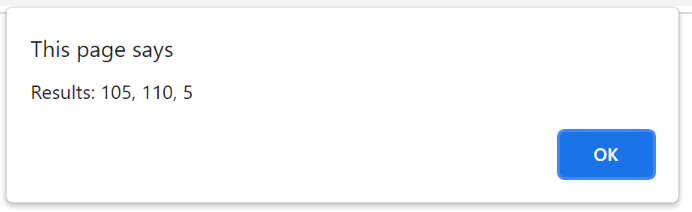# How to Round off a number to next multiple of 5 using JavaScript?

In this tutorial, we will learn how to round off a number to next multiple of 5 using JavaScript? By IncludeHelp Last updated : July 30, 2023

In this problem, we are given a floating-point number. Our task is to find a number that is round off of the number but is a multiple of 5.

Here, we will find the nearest multiple of 5 of the given number.

Here, are some problems, that will make the problem clearer.

```Input: 43.56
Output: 45

Input: 21
Output: 25
```

Solving such problems involves the use of methods of math library in javascript. Using rounding methods of the division of the number by 5 and then multiply the rounded number by 5.

## Method 1: Using Math.ceil() method

The math.ceil() method is used to return the nearest integer greater than the given integer.

We will first divide the number by 5, then pass the value to ceil method and multiply the output of by 5 to get the result.

### Example

```<script>
var num = 3.4121;
alert("nearest multiple of 5 of the number is : "+
(Math.ceil(num / 5) * 5));
</script>
```

Output:## Method 2: Using Math.floor() method

In this method, check whether the given number is divisible by 5 or not, if the number is not divisible by 5, then divide the number by 5 and take the floor value and again multiply the number by 5 and add 5 as well.

### Example

```<script>
var x = 105;
var result1 = 0;
var result2 =0;
var result3=0;

if (x % 5 == 0) {
result1 = Math.floor(x / 5) * 5;
} else {
result1 = (Math.floor(x / 5) * 5) + 5;
}

x = 108;
if (x % 5 == 0) {
result2 = Math.floor(x / 5) * 5;
} else {
result2 = (Math.floor(x / 5) * 5) + 5;
}

x = 1;
if (x % 5 == 0) {
result3 = Math.floor(x / 5) * 5;
} else {
result3 = (Math.floor(x / 5) * 5) + 5;
}

alert("Results: " + result1 + ", " + result2 + ", " + result3);
</script>
```

Output: